# Maharashtra State HSC Board Question Paper for Class 12th Maths 2019 In PDF

## MSBSHSE HSC Board 12th Class Question Paper Mathematics English Medium 2019 with Solutions – Free Download

Maharashtra State Board Class 12th maths 2019 question paper with solutions are available on this page, by BYJU’S, in downloadable pdf format and also in the text for the students to prepare well for their exams. The page also consists of Maths Question Paper 2019 Class 12, which acts as an extra resource. Students are able to access all the Maharashtra HSC Board previous year maths question papers here. Students can analyse their confidence and preparation level for the upcoming final exams and also ace it by practising these papers.

MSBSHSE Class 12th Maths Question Paper With Solutions 2019
QUESTION PAPER CODE J – 60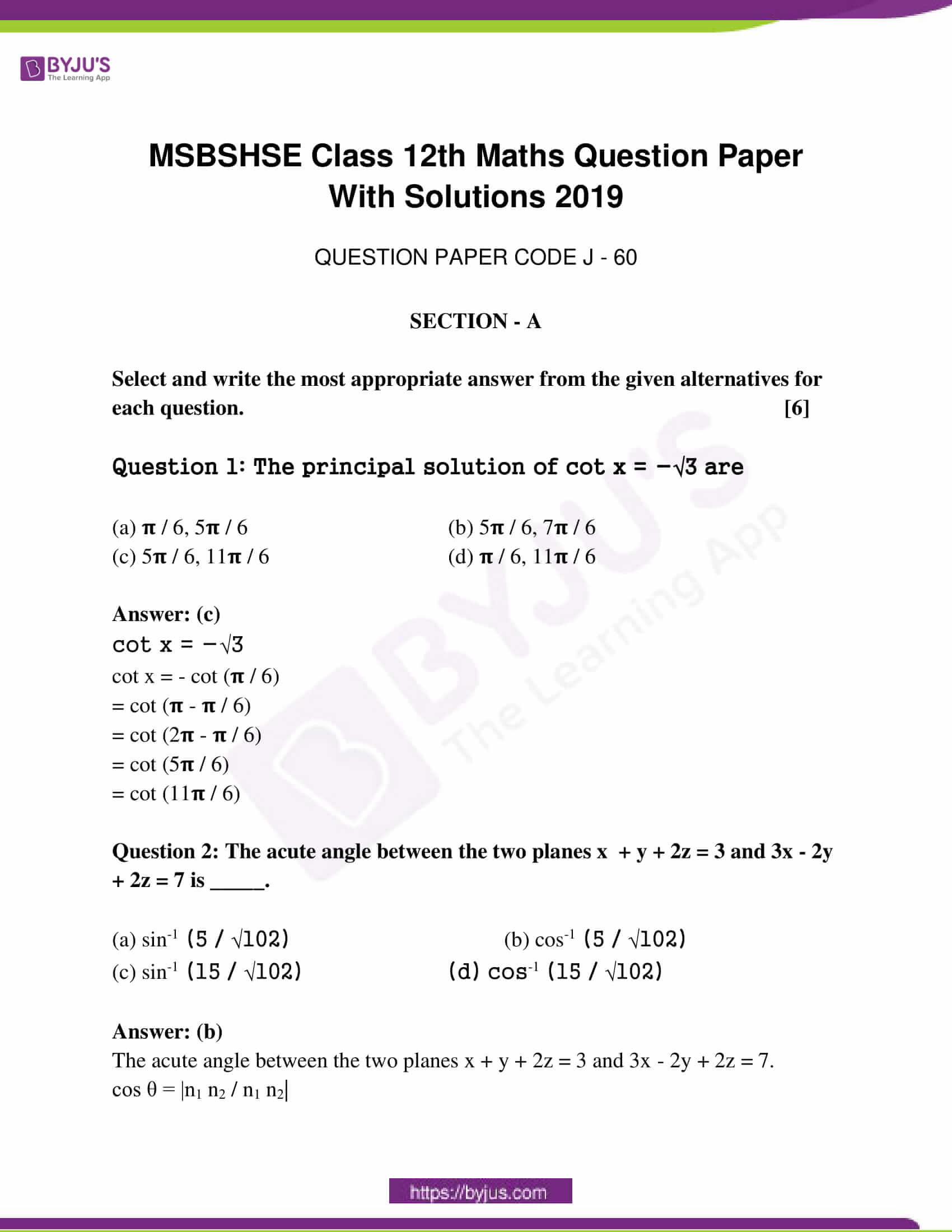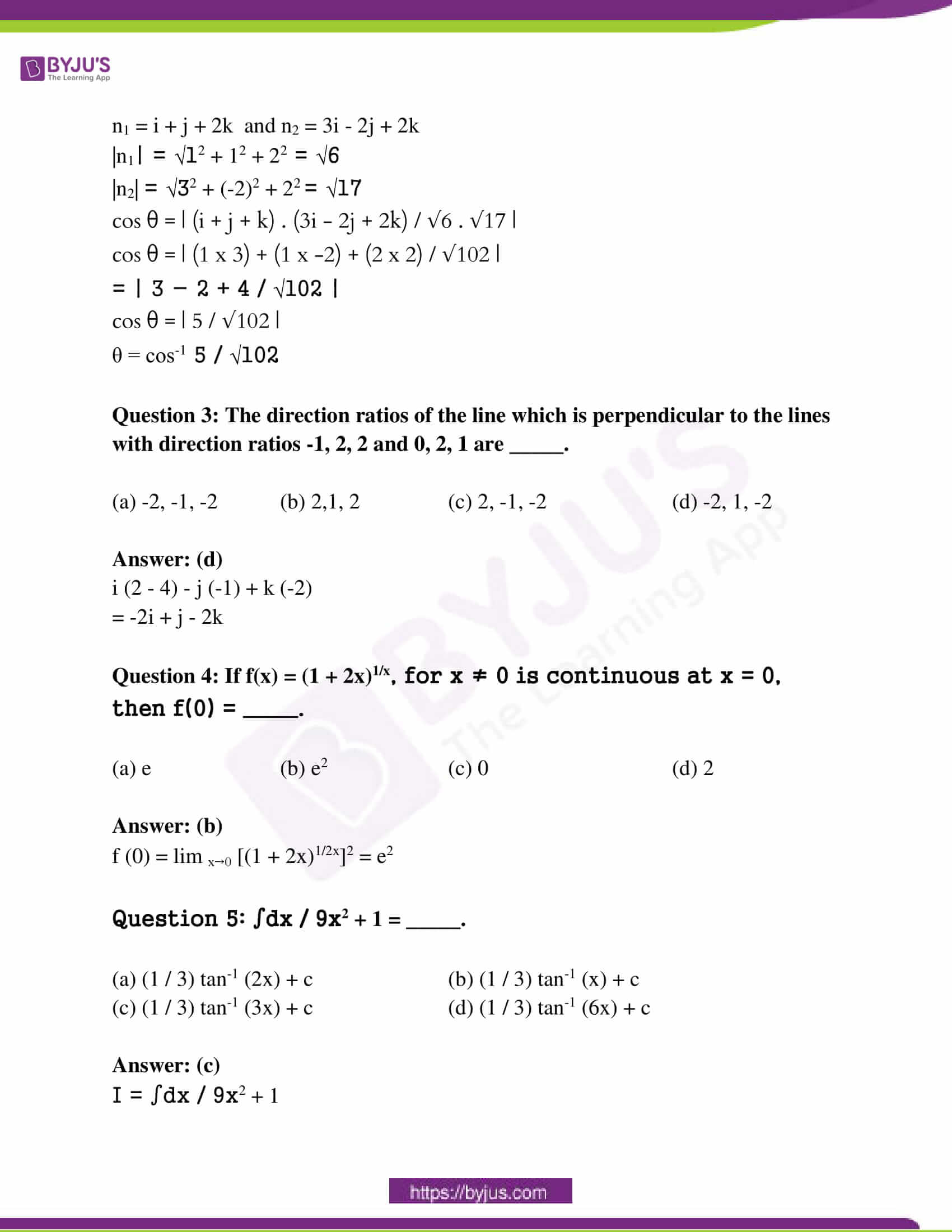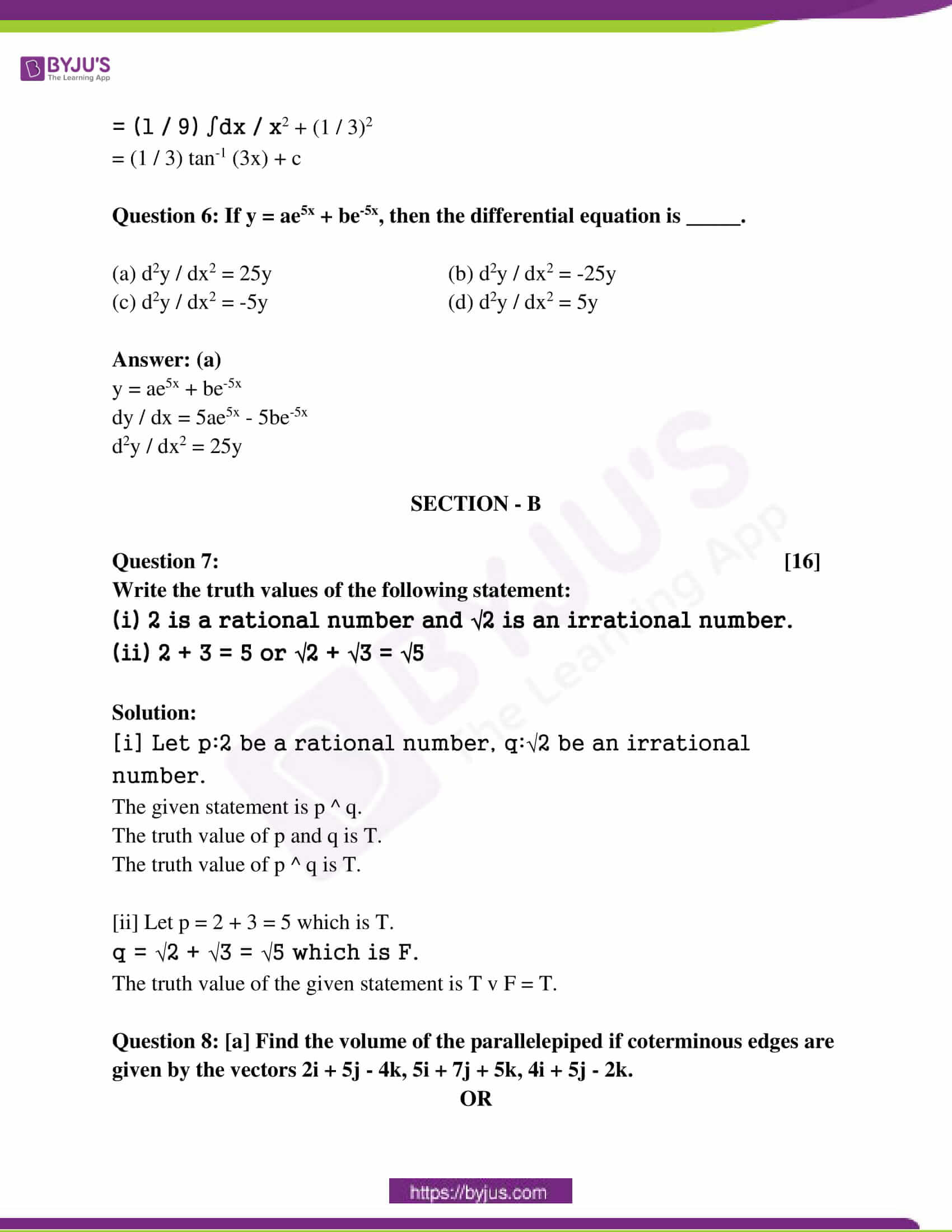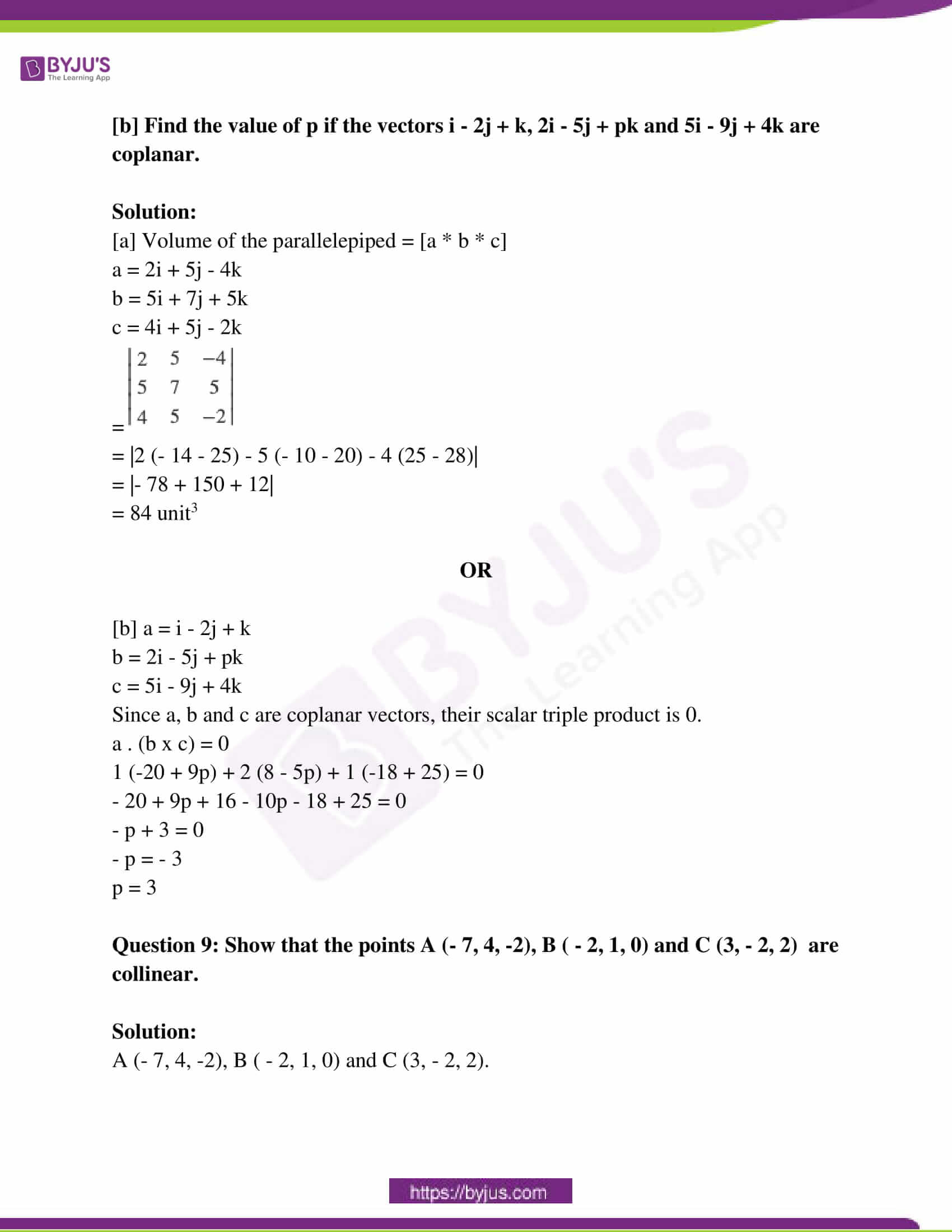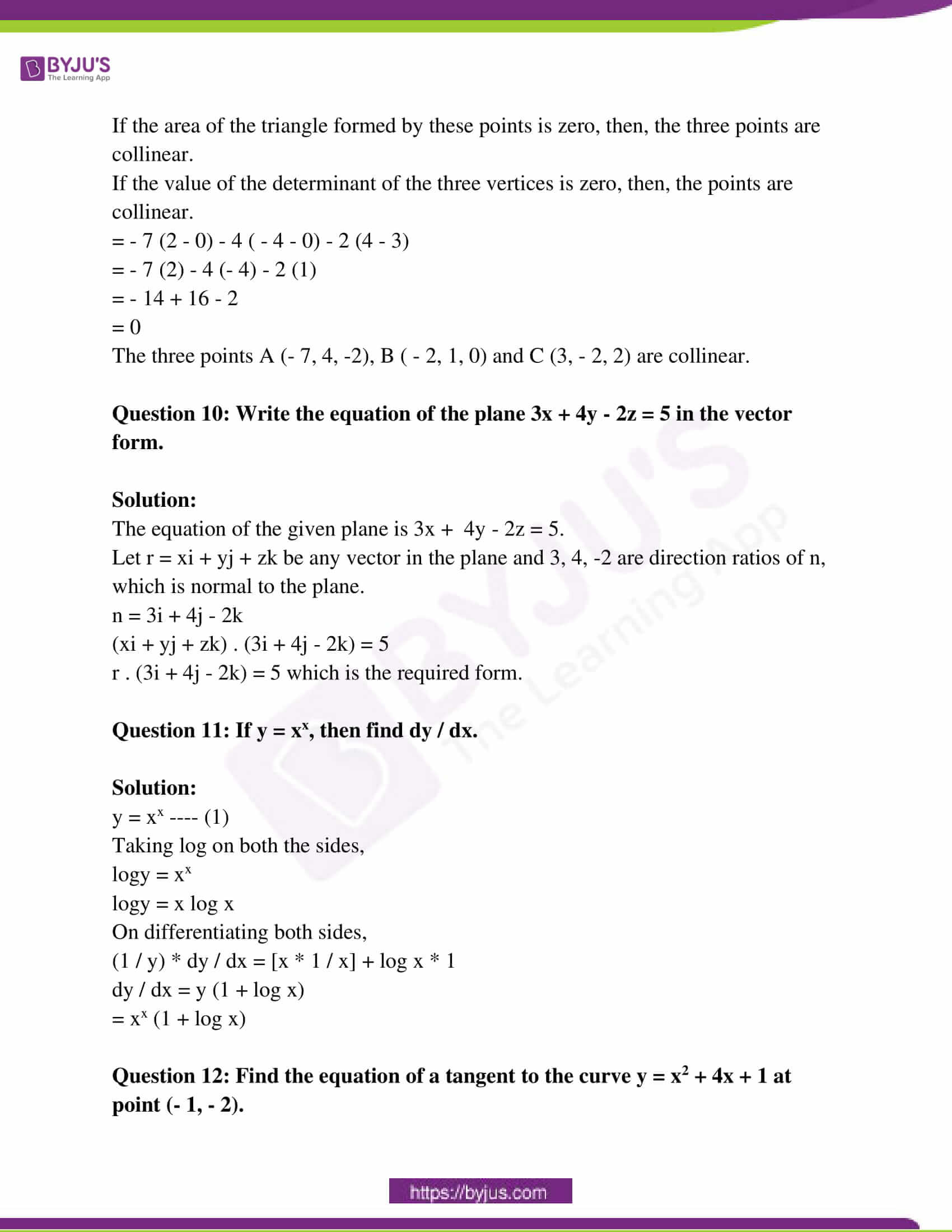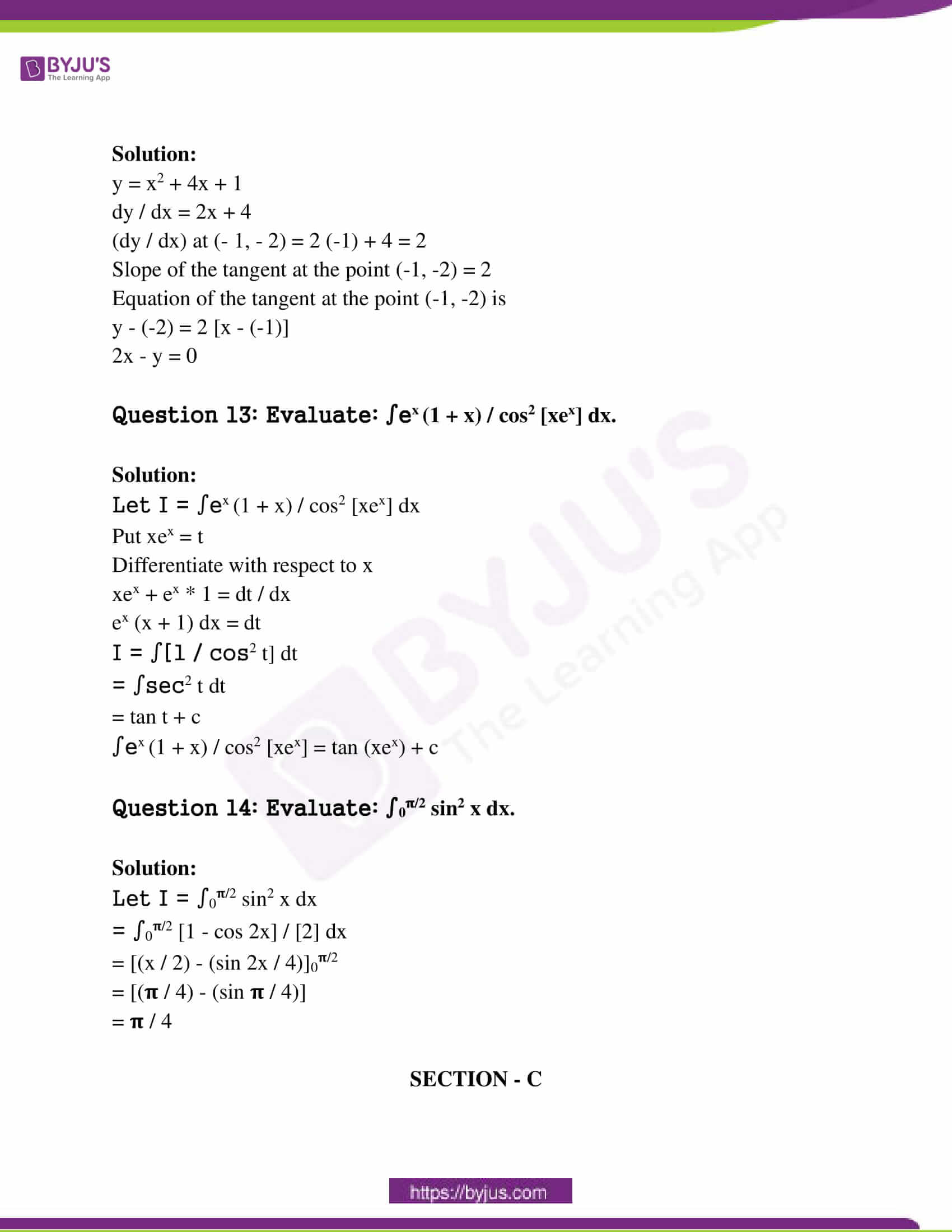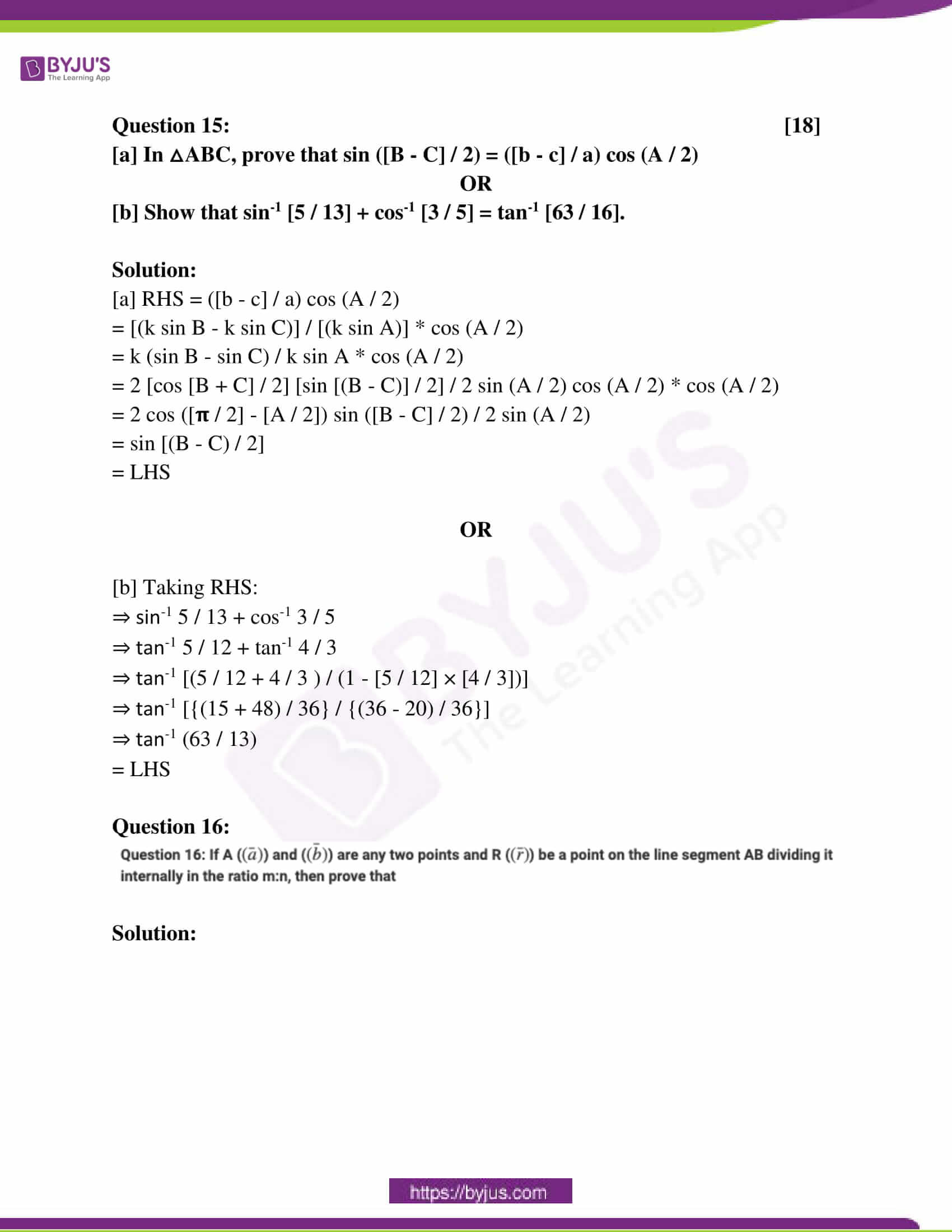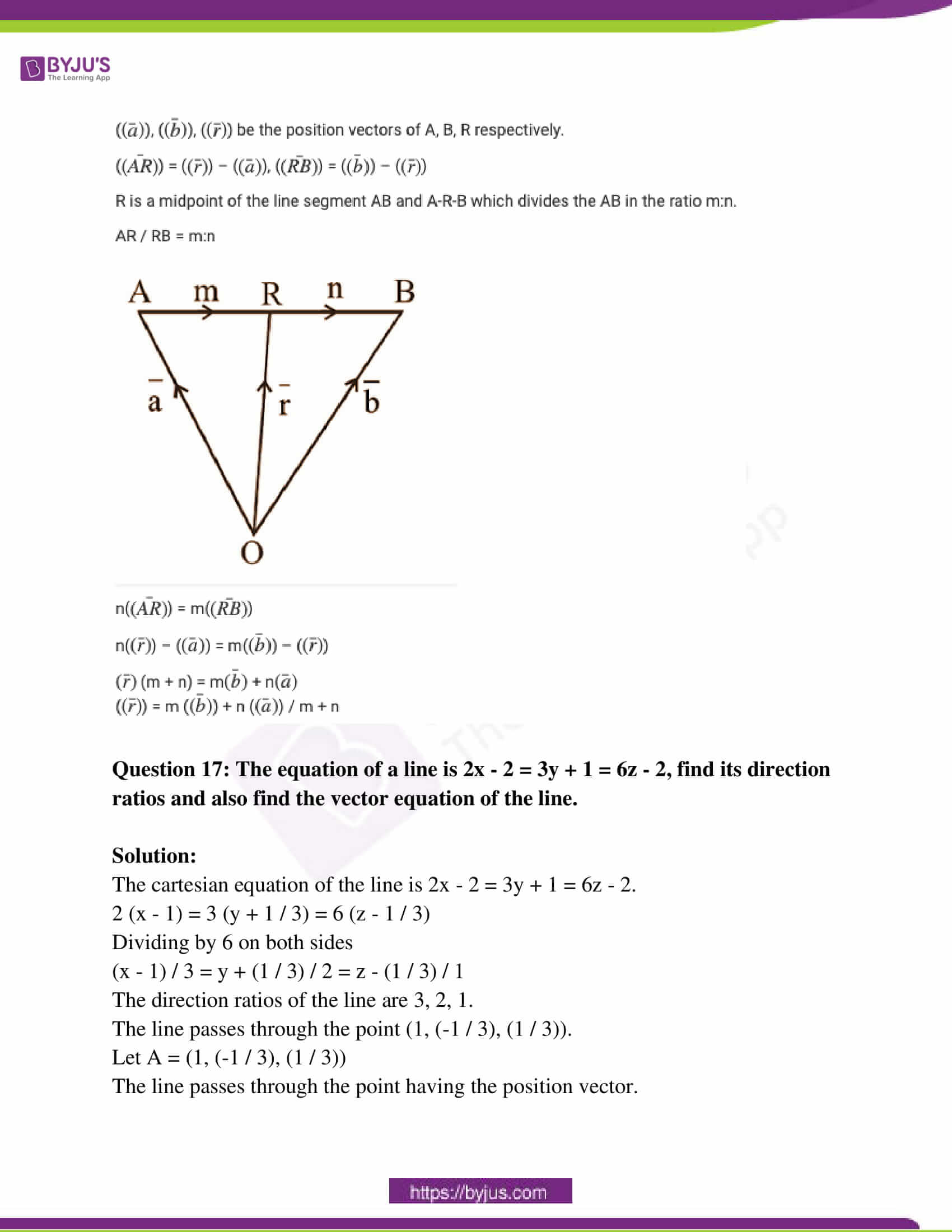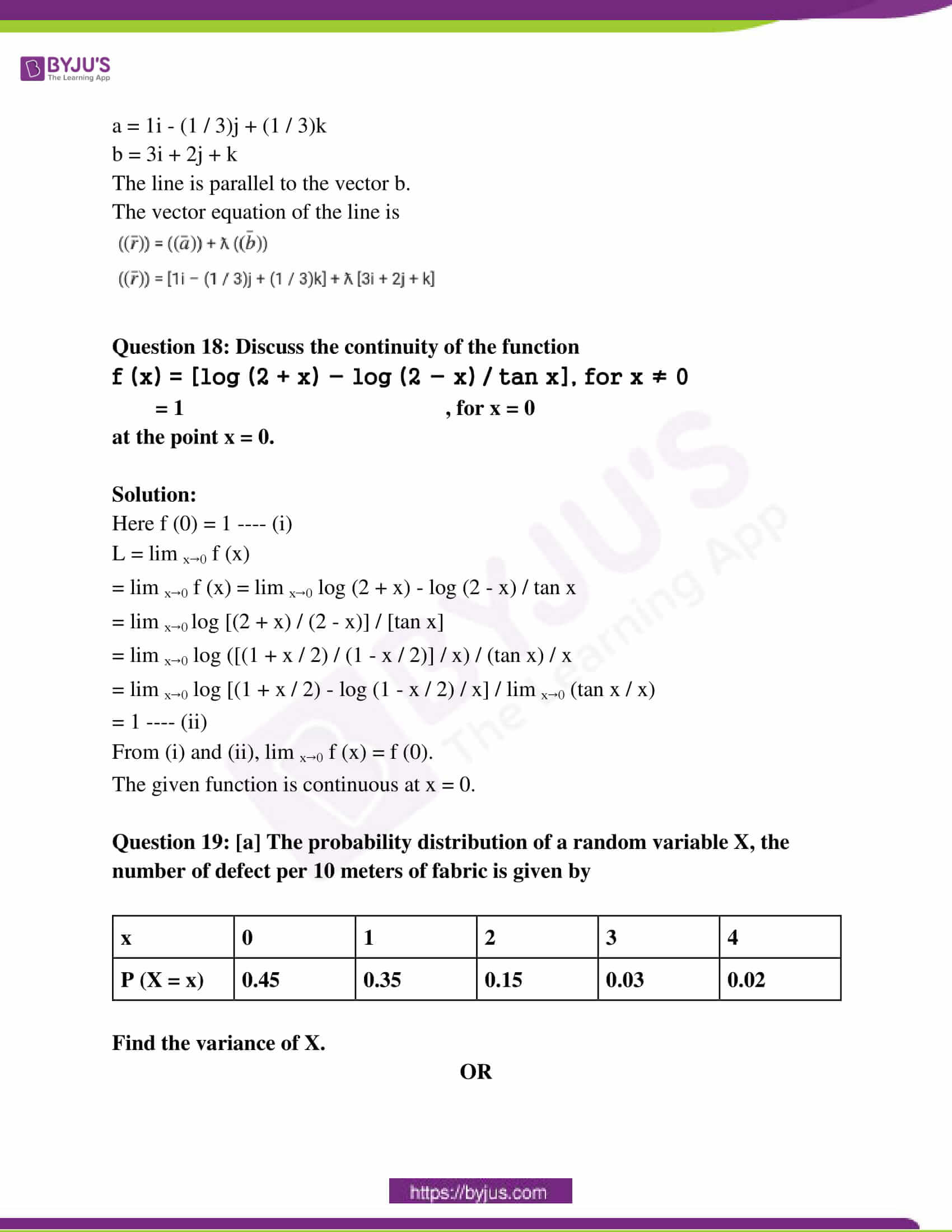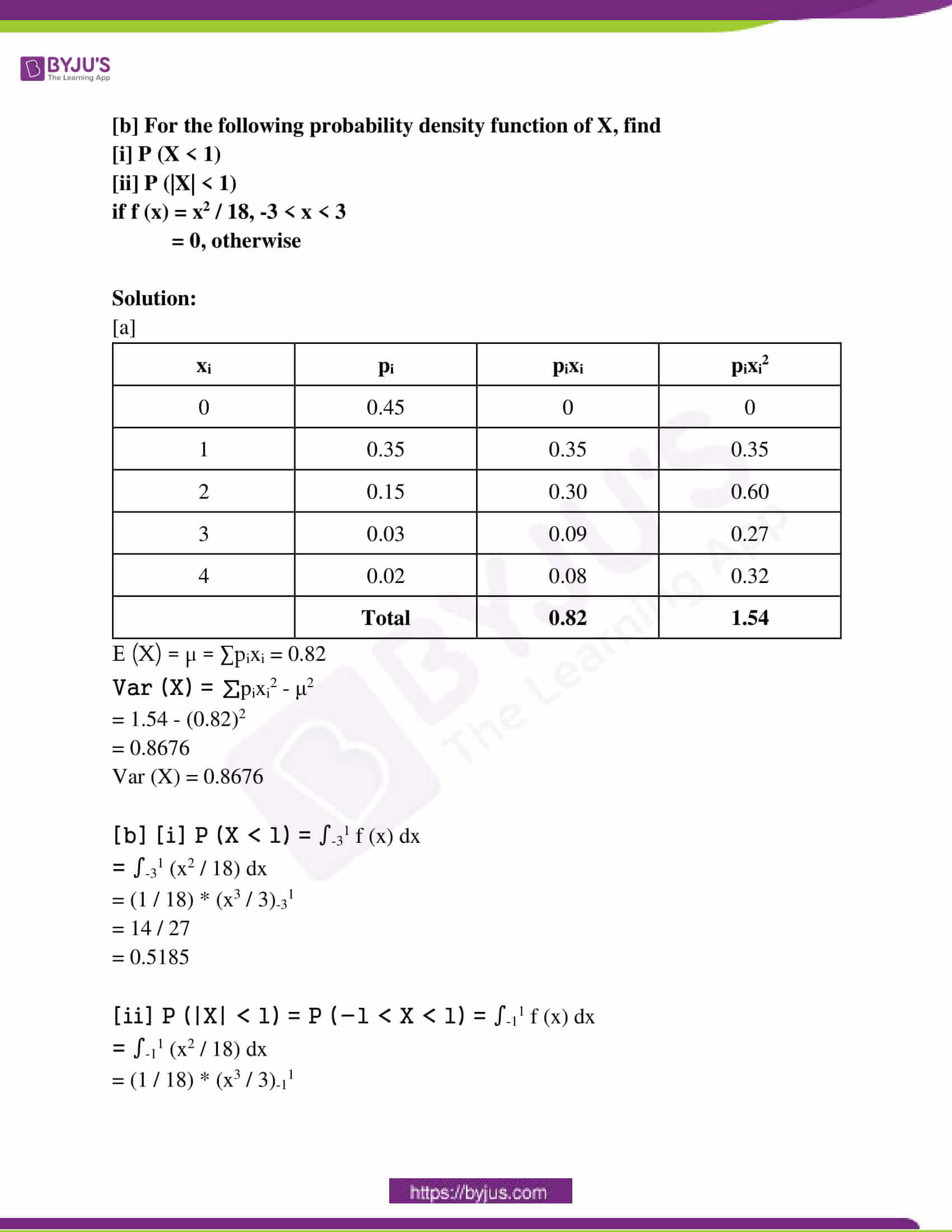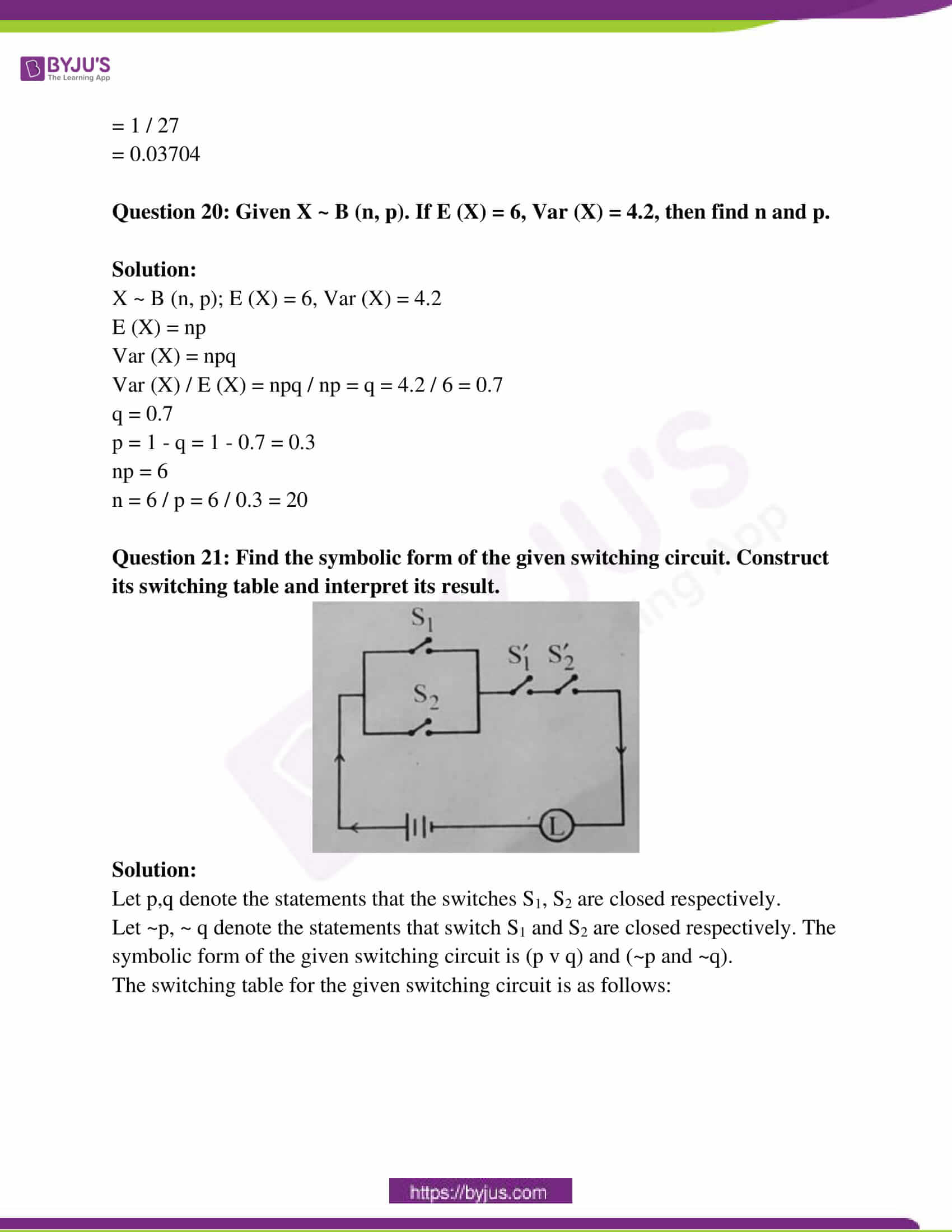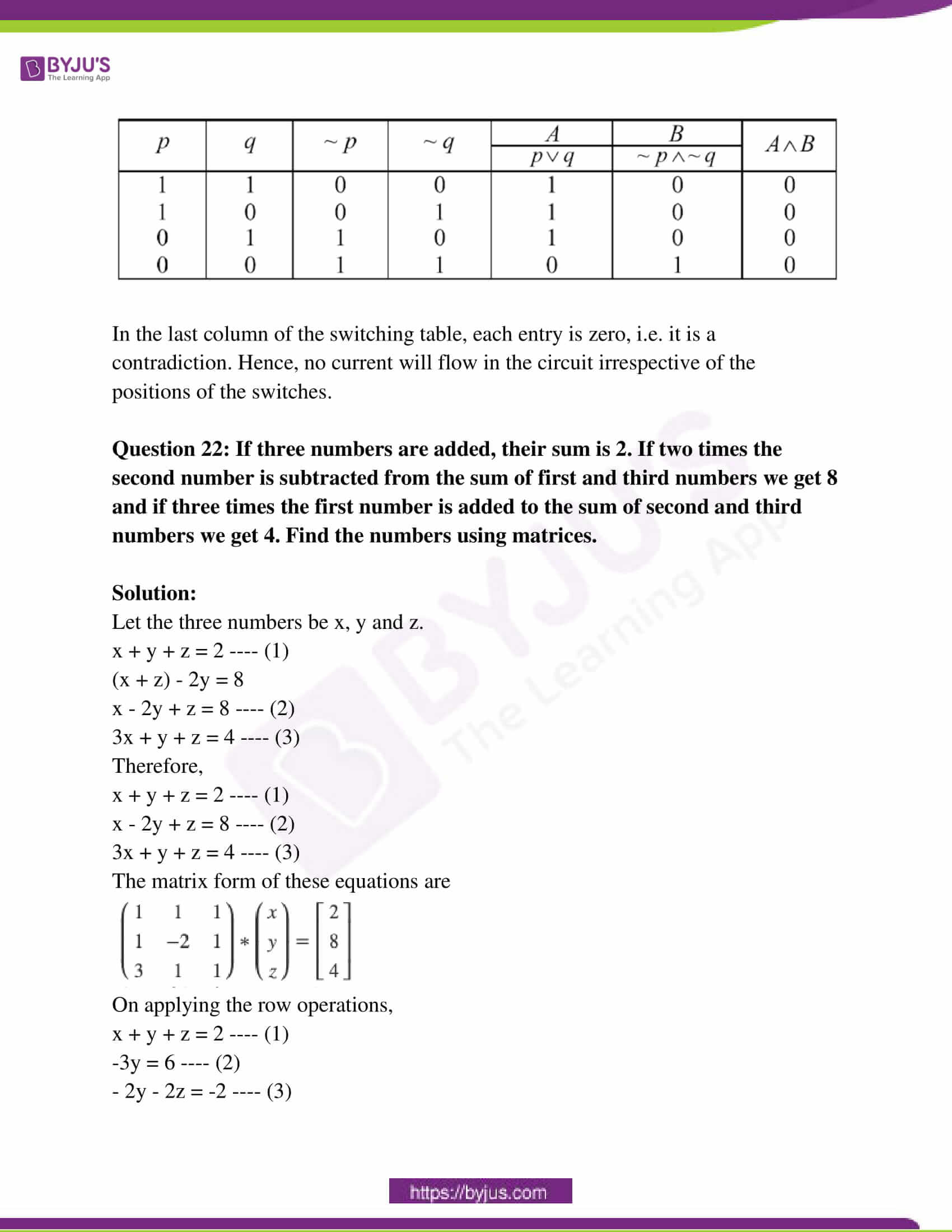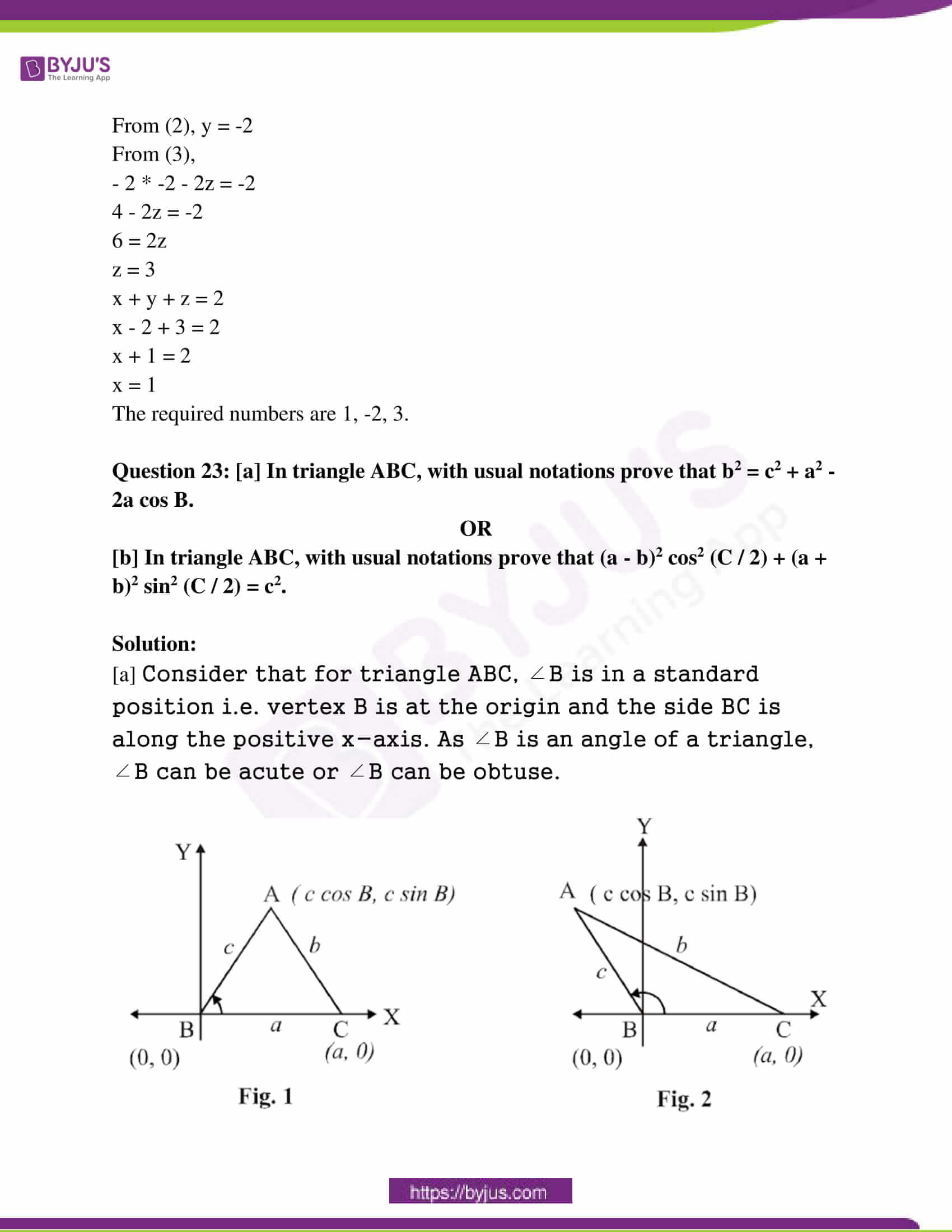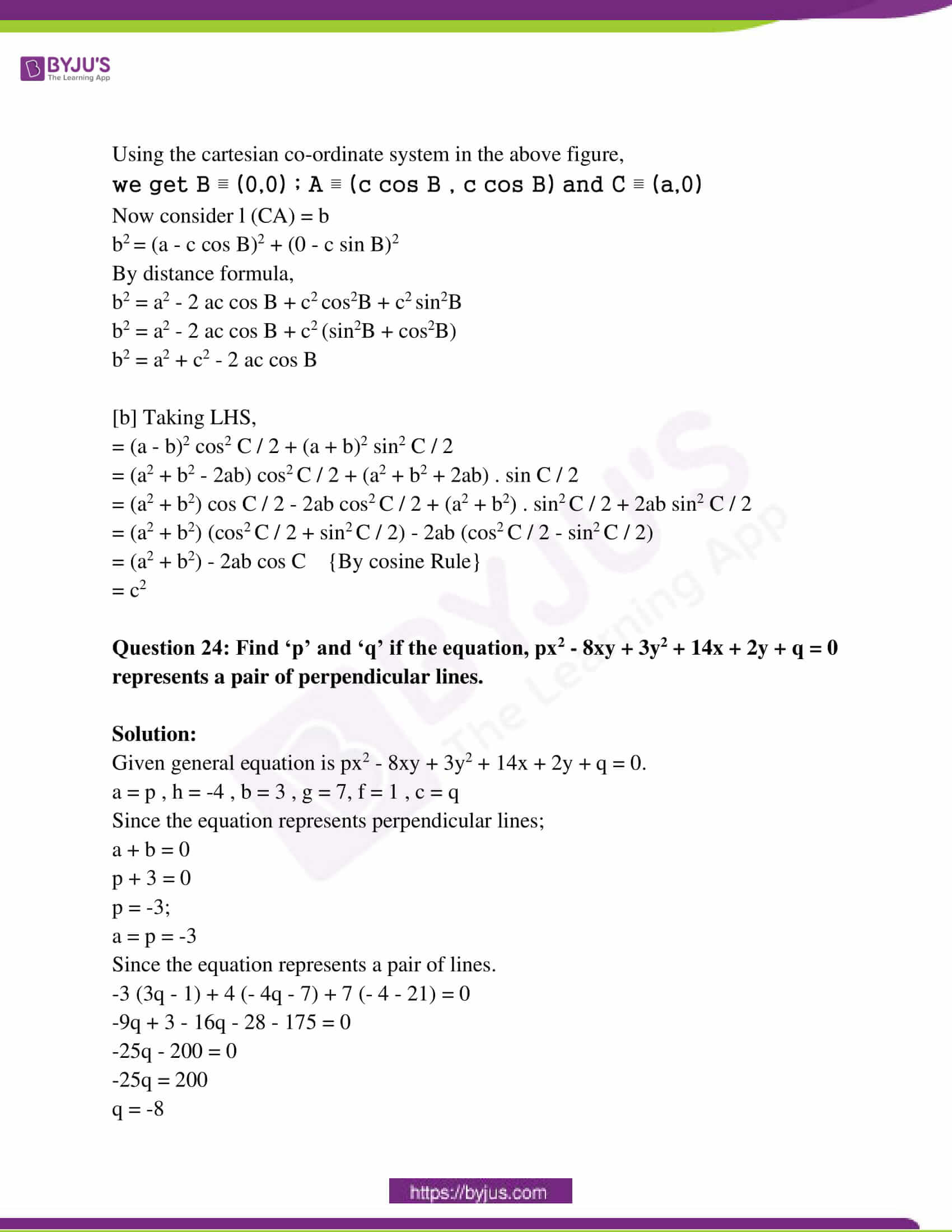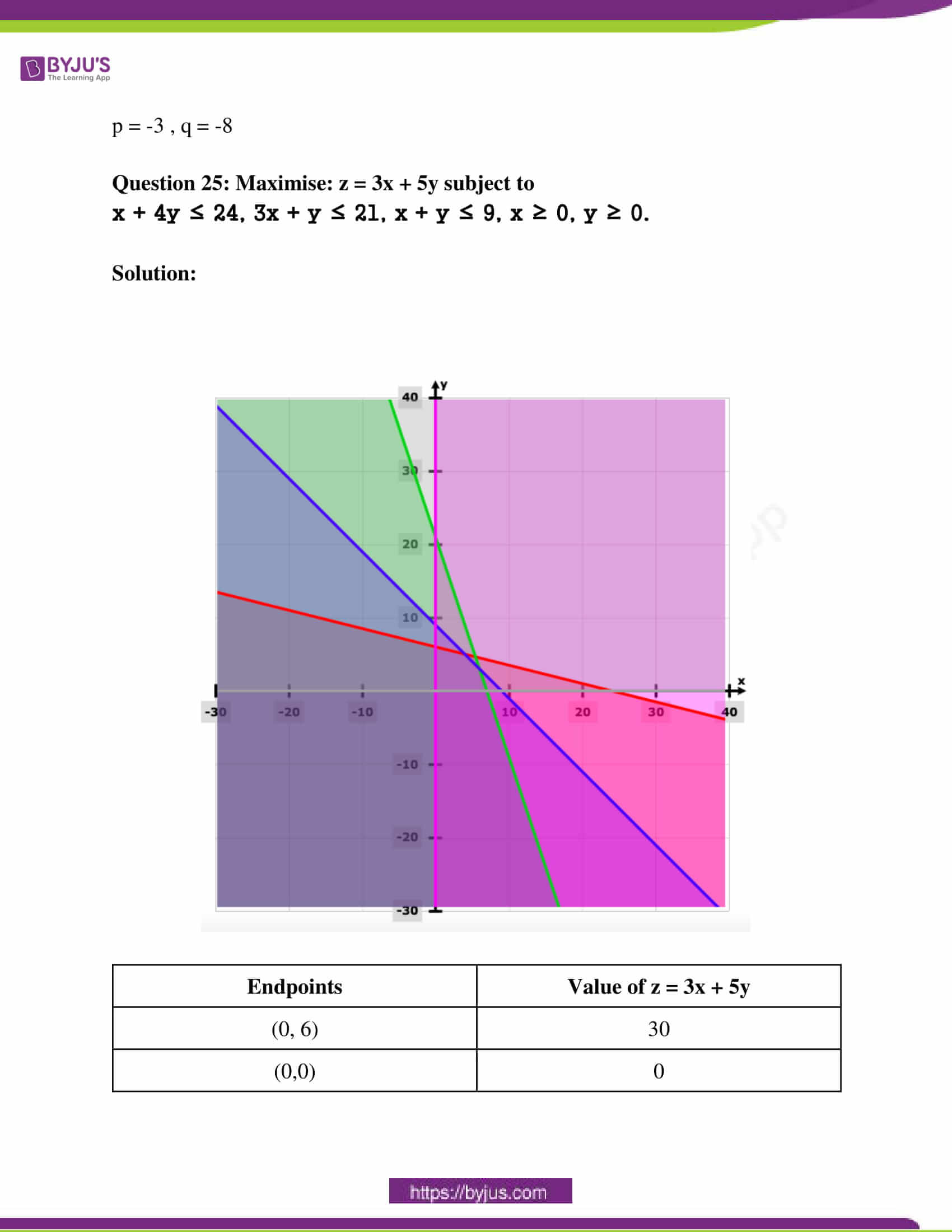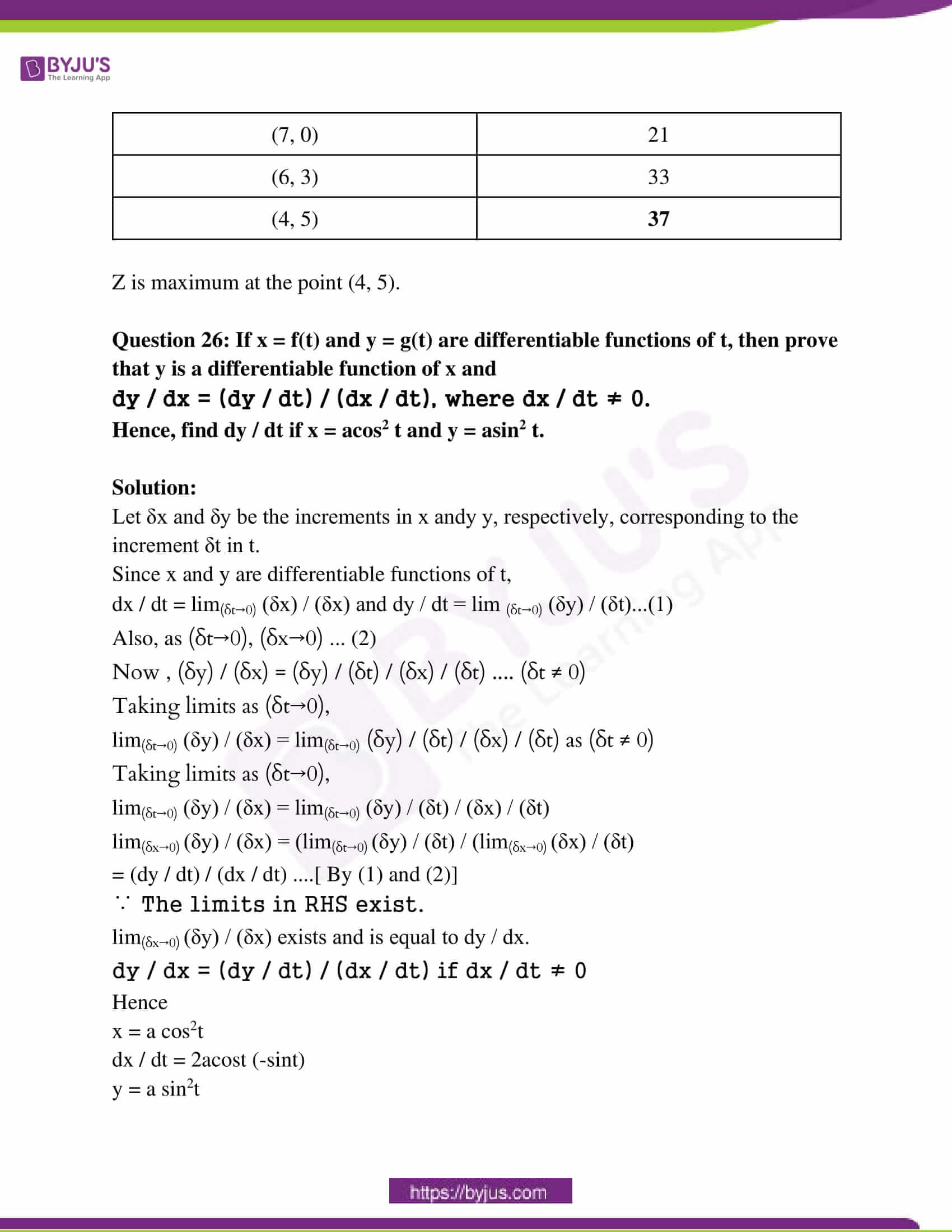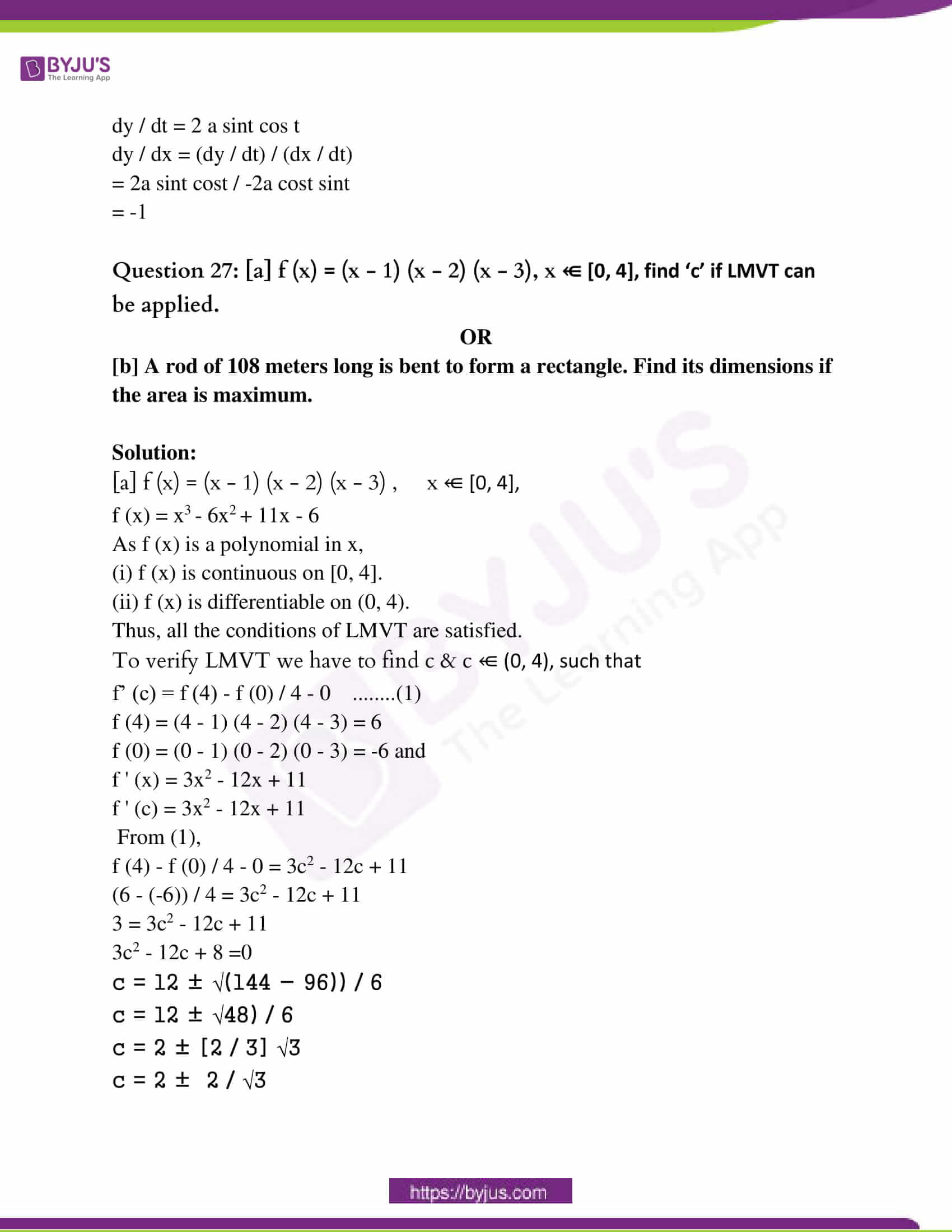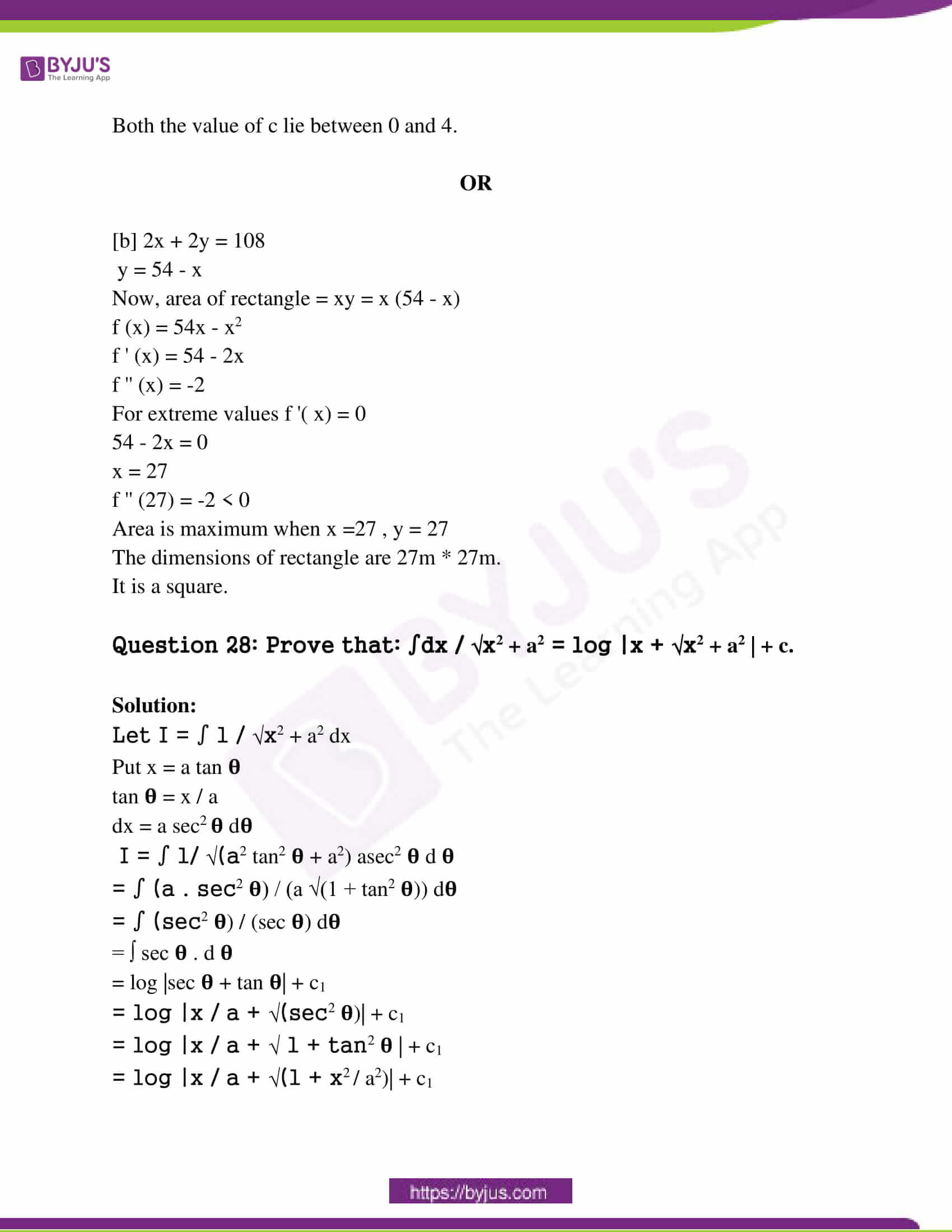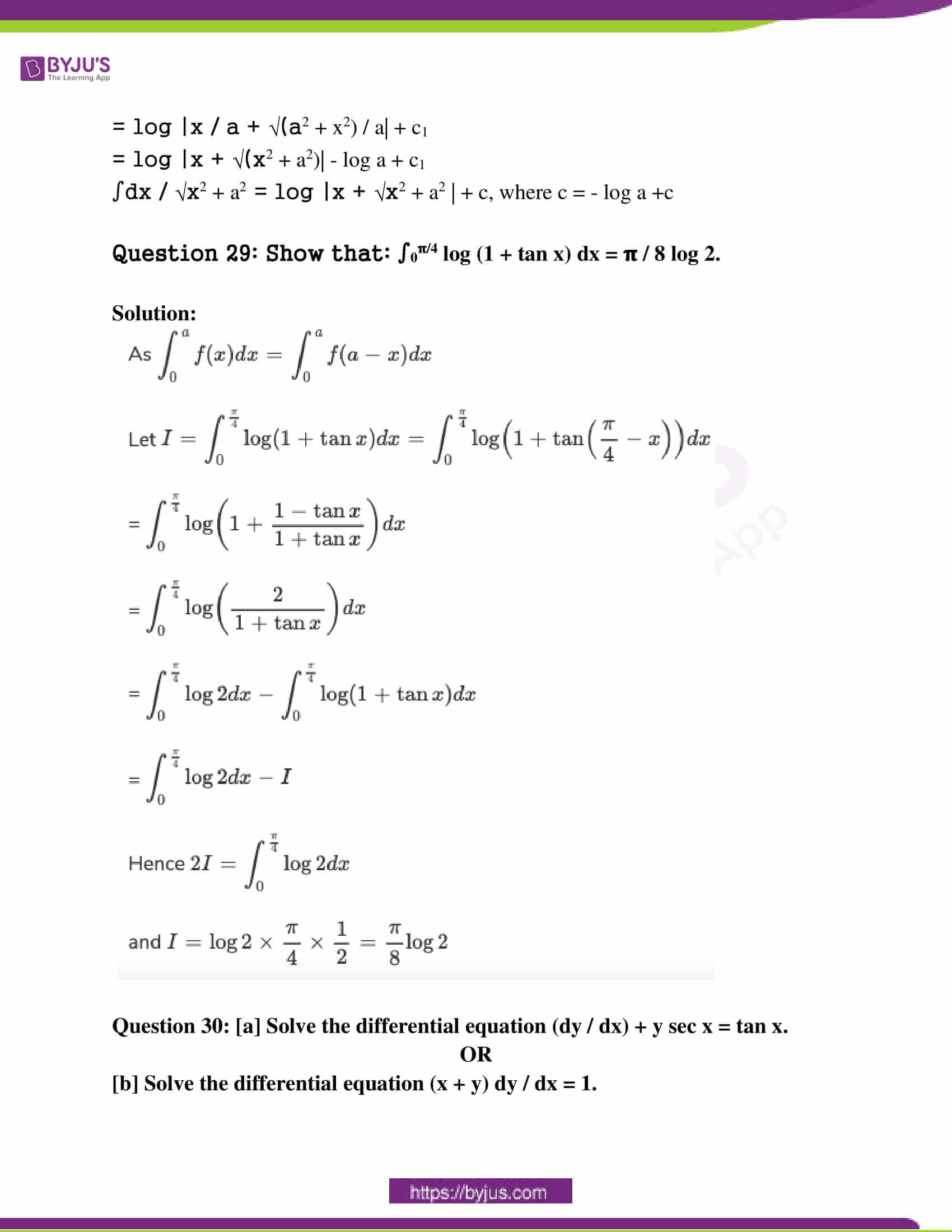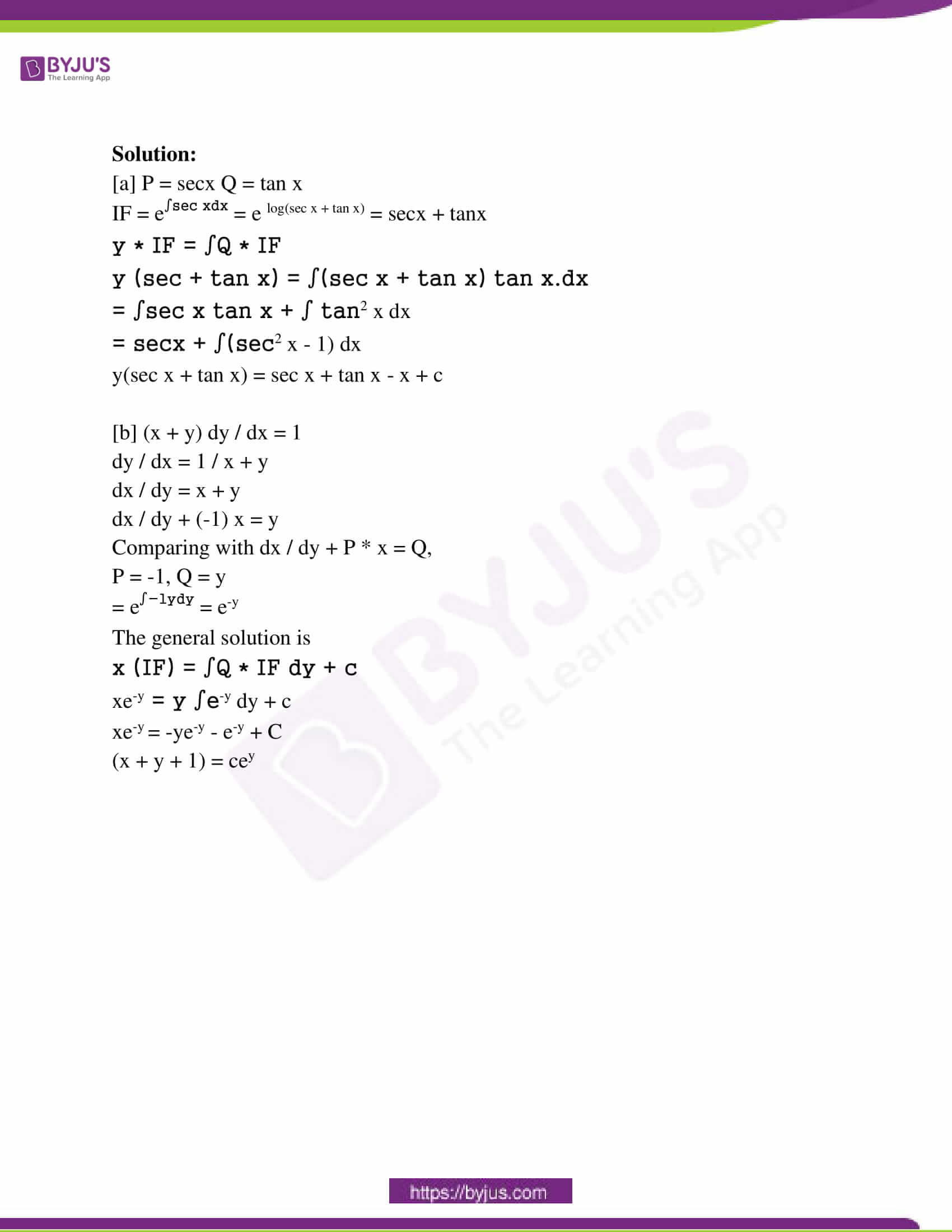SECTION – A

Select and write the most appropriate answer from the given alternatives for each question. 

Question 1: The principal solution of cot x = -√3 are

(a) 𝛑 / 6, 5𝛑 / 6 (b) 5𝛑 / 6, 7𝛑 / 6

(c) 5𝛑 / 6, 11𝛑 / 6 (d) 𝛑 / 6, 11𝛑 / 6

cot x = -√3

cot x = – cot (𝛑 / 6)

= cot (𝛑 – 𝛑 / 6)

= cot (2𝛑 – 𝛑 / 6)

= cot (5𝛑 / 6)

= cot (11𝛑 / 6)

Question 2: The acute angle between the two planes x + y + 2z = 3 and 3x – 2y + 2z = 7 is _____.

(a) sin-1 (5 / √102) (b) cos-1 (5 / √102)

(c) sin-1 (15 / √102) (d) cos-1 (15 / √102)

The acute angle between the two planes x + y + 2z = 3 and 3x – 2y + 2z = 7.

cos θ = |n1 n2 / n1 n2|

n1 = i + j + 2k and n2 = 3i – 2j + 2k

|n1| = √12 + 12 + 22 = √6

|n2| = √32 + (-2)2 + 22 = √17

cos θ = | (i + j + k) . (3i – 2j + 2k) / √6 . √17 |

cos θ = | (1 x 3) + (1 x -2) + (2 x 2) / √102 |

= | 3 – 2 + 4 / √102 |

cos θ = | 5 / √102 |

θ = cos-1 5 / √102

Question 3: The direction ratios of the line which is perpendicular to the lines with direction ratios -1, 2, 2 and 0, 2, 1 are _____.

(a) -2, -1, -2 (b) 2,1, 2 (c) 2, -1, -2 (d) -2, 1, -2

i (2 – 4) – j (-1) + k (-2)

= -2i + j – 2k

Question 4: If f(x) = (1 + 2x)1/x, for x ≠ 0 is continuous at x = 0, then f(0) = _____.

(a) e (b) e2 (c) 0 (d) 2

f (0) = lim x→0 [(1 + 2x)1/2x]2 = e2

Question 5: ∫dx / 9x2 + 1 = _____.

(a) (1 / 3) tan-1 (2x) + c (b) (1 / 3) tan-1 (x) + c

(c) (1 / 3) tan-1 (3x) + c (d) (1 / 3) tan-1 (6x) + c

I = ∫dx / 9x2 + 1

= (1 / 9) ∫dx / x2 + (1 / 3)2

= (1 / 3) tan-1 (3x) + c

Question 6: If y = ae5x + be-5x, then the differential equation is _____.

(a) d2y / dx2 = 25y (b) d2y / dx2 = -25y

(c) d2y / dx2 = -5y (d) d2y / dx2 = 5y

y = ae5x + be-5x

dy / dx = 5ae5x – 5be-5x

d2y / dx2 = 25y

SECTION – B

Question 7: 

Write the truth values of the following statement:

(i) 2 is a rational number and √2 is an irrational number.

(ii) 2 + 3 = 5 or √2 + √3 = √5

Solution:

[i] Let p:2 be a rational number, q:√2 be an irrational number.

The given statement is p ^ q.

The truth value of p and q is T.

The truth value of p ^ q is T.

[ii] Let p = 2 + 3 = 5 which is T.

q = √2 + √3 = √5 which is F.

The truth value of the given statement is T v F = T.

Question 8: [a] Find the volume of the parallelepiped if coterminous edges are given by the vectors 2i + 5j – 4k, 5i + 7j + 5k, 4i + 5j – 2k.

OR

[b] Find the value of p if the vectors i – 2j + k, 2i – 5j + pk and 5i – 9j + 4k are coplanar.

Solution:

[a] Volume of the parallelepiped = [a * b * c]

a = 2i + 5j – 4k

b = 5i + 7j + 5k

c = 4i + 5j – 2k

$$\begin{array}{l}=\left|\begin{array}{ccc}2&5&-4\\5&7&5\\4&5&-2\end{array}\right|\\\end{array}$$
\\

= |2 (- 14 – 25) – 5 (- 10 – 20) – 4 (25 – 28)|

= |- 78 + 150 + 12|

= 84 unit3

OR

[b] a = i – 2j + k

b = 2i – 5j + pk

c = 5i – 9j + 4k

Since a, b and c are coplanar vectors, their scalar triple product is 0.

a . (b x c) = 0

1 (-20 + 9p) + 2 (8 – 5p) + 1 (-18 + 25) = 0

– 20 + 9p + 16 – 10p – 18 + 25 = 0

– p + 3 = 0

– p = – 3

p = 3

Question 9: Show that the points A (- 7, 4, -2), B ( – 2, 1, 0) and C (3, – 2, 2) are collinear.

Solution:

A (- 7, 4, -2), B ( – 2, 1, 0) and C (3, – 2, 2).

If the area of the triangle formed by these points is zero, then, the three points are collinear.

If the value of the determinant of the three vertices is zero, then, the points are collinear.

= – 7 (2 – 0) – 4 ( – 4 – 0) – 2 (4 – 3)

= – 7 (2) – 4 (- 4) – 2 (1)

= – 14 + 16 – 2

= 0

The three points A (- 7, 4, -2), B ( – 2, 1, 0) and C (3, – 2, 2) are collinear.

Question 10: Write the equation of the plane 3x + 4y – 2z = 5 in the vector form.

Solution:

The equation of the given plane is 3x + 4y – 2z = 5.

Let r = xi + yj + zk be any vector in the plane and 3, 4, -2 are direction ratios of n, which is normal to the plane.

n = 3i + 4j – 2k

(xi + yj + zk) . (3i + 4j – 2k) = 5

r . (3i + 4j – 2k) = 5 which is the required form.

Question 11: If y = xx, then find dy / dx.

Solution:

y = xx —- (1)

Taking log on both the sides,

logy = xx

logy = x log x

On differentiating both sides,

(1 / y) * dy / dx = [x * 1 / x] + log x * 1

dy / dx = y (1 + log x)

= xx (1 + log x)

Question 12: Find the equation of a tangent to the curve y = x2 + 4x + 1 at point (- 1, – 2).

Solution:

y = x2 + 4x + 1

dy / dx = 2x + 4

(dy / dx) at (- 1, – 2) = 2 (-1) + 4 = 2

Slope of the tangent at the point (-1, -2) = 2

Equation of the tangent at the point (-1, -2) is

y – (-2) = 2 [x – (-1)]

2x – y = 0

Question 13: Evaluate: ∫ex (1 + x) / cos2 [xex] dx.

Solution:

Let I = ∫ex (1 + x) / cos2 [xex] dx

Put xex = t

Differentiate with respect to x

xex + ex * 1 = dt / dx

ex (x + 1) dx = dt

I = ∫[1 / cos2 t] dt

= ∫sec2 t dt

= tan t + c

∫ex (1 + x) / cos2 [xex] = tan (xex) + c

Question 14: Evaluate: ∫0𝛑/2 sin2 x dx.

Solution:

Let I = ∫0𝛑/2 sin2 x dx

= ∫0𝛑/2 [1 – cos 2x] /  dx

= [(x / 2) – (sin 2x / 4)]0𝛑/2

= [(𝛑 / 4) – (sin 𝛑 / 4)]

= 𝛑 / 4

SECTION – C

Question 15: 

[a] In △ABC, prove that sin ([B – C] / 2) = ([b – c] / a) cos (A / 2)

OR

[b] Show that sin-1 [5 / 13] + cos-1 [3 / 5] = tan-1 [63 / 16].

Solution:

[a] RHS = ([b – c] / a) cos (A / 2)

= [(k sin B – k sin C)] / [(k sin A)] * cos (A / 2)

= k (sin B – sin C) / k sin A * cos (A / 2)

= 2 [cos [B + C] / 2] [sin [(B – C)] / 2] / 2 sin (A / 2) cos (A / 2) * cos (A / 2)

= 2 cos ([𝛑 / 2] – [A / 2]) sin ([B – C] / 2) / 2 sin (A / 2)

= sin [(B – C) / 2]

= LHS

OR

[b] Taking RHS:

⇒ sin-1 5 / 13 + cos-1 3 / 5

⇒ tan-1 5 / 12 + tan-1 4 / 3

⇒ tan-1 [(5 / 12 + 4 / 3 ) / (1 – [5 / 12] × [4 / 3])]

⇒ tan-1 [{(15 + 48) / 36} / {(36 – 20) / 36}]

⇒ tan-1 (63 / 13)

= LHS

Question 16: If A (

$$\begin{array}{l}(\bar {a})\end{array}$$
) and (
$$\begin{array}{l}(\bar {b})\end{array}$$
) are any two points and R (
$$\begin{array}{l}(\bar {r})\end{array}$$
) be a point on the line segment AB dividing it internally in the ratio m:n, then prove that

(

$$\begin{array}{l}(\bar {r})\end{array}$$
) = m (
$$\begin{array}{l}(\bar {b})\end{array}$$
) + n (
$$\begin{array}{l}(\bar {a})\end{array}$$
) / m + n.

Solution:

(

$$\begin{array}{l}(\bar {a})\end{array}$$
), (
$$\begin{array}{l}(\bar {b})\end{array}$$
), (
$$\begin{array}{l}(\bar {r})\end{array}$$
) be the position vectors of A, B, R respectively.

(

$$\begin{array}{l}(\bar {AR})\end{array}$$
) = (
$$\begin{array}{l}(\bar {r})\end{array}$$
) – (
$$\begin{array}{l}(\bar {a})\end{array}$$
), (
$$\begin{array}{l}(\bar {RB})\end{array}$$
) = (
$$\begin{array}{l}(\bar {b})\end{array}$$
) – (
$$\begin{array}{l}(\bar {r})\end{array}$$
)

R is a midpoint of the line segment AB and A-R-B which divides the AB in the ratio m:n.

AR / RB = m:n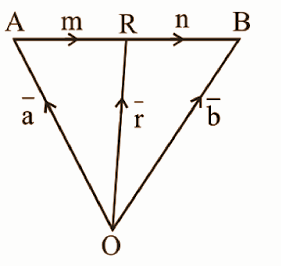n(

$$\begin{array}{l}(\bar {AR})\end{array}$$
) = m(
$$\begin{array}{l}(\bar {RB})\end{array}$$
)

n(

$$\begin{array}{l}(\bar {r})\end{array}$$
) – (
$$\begin{array}{l}(\bar {a})\end{array}$$
) = m(
$$\begin{array}{l}(\bar {b})\end{array}$$
) – (
$$\begin{array}{l}(\bar {r})\end{array}$$
)

$$\begin{array}{l}(\bar {r})\end{array}$$
(m + n) = m
$$\begin{array}{l}(\bar {b})\end{array}$$
+ n
$$\begin{array}{l}(\bar {a})\end{array}$$

(

$$\begin{array}{l}(\bar {r})\end{array}$$
) = m (
$$\begin{array}{l}(\bar {b})\end{array}$$
) + n (
$$\begin{array}{l}(\bar {a})\end{array}$$
) / m + n

Question 17: The equation of a line is 2x – 2 = 3y + 1 = 6z – 2, find its direction ratios and also find the vector equation of the line.

Solution:

The cartesian equation of the line is 2x – 2 = 3y + 1 = 6z – 2.

2 (x – 1) = 3 (y + 1 / 3) = 6 (z – 1 / 3)

Dividing by 6 on both sides

(x – 1) / 3 = y + (1 / 3) / 2 = z – (1 / 3) / 1

The direction ratios of the line are 3, 2, 1.

The line passes through the point (1, (-1 / 3), (1 / 3)).

Let A = (1, (-1 / 3), (1 / 3))

The line passes through the point having the position vector.

a = 1i – (1 / 3)j + (1 / 3)k

b = 3i + 2j + k

The line is parallel to the vector b.

The vector equation of the line is

(

$$\begin{array}{l}(\bar {r})\end{array}$$
) = (
$$\begin{array}{l}(\bar {a})\end{array}$$
) + ƛ (
$$\begin{array}{l}(\bar {b})\end{array}$$
)

(

$$\begin{array}{l}(\bar {r})\end{array}$$
) = [1i – (1 / 3)j + (1 / 3)k] + ƛ [3i + 2j + k]

Question 18: Discuss the continuity of the function

f (x) = [log (2 + x) – log (2 – x) / tan x], for x ≠ 0

= 1 , for x = 0

at the point x = 0.

Solution:

Here f (0) = 1 —- (i)

L = lim x→0 f (x)

= lim x→0 f (x) = lim x→0 log (2 + x) – log (2 – x) / tan x

= lim x→0 log [(2 + x) / (2 – x)] / [tan x]

= lim x→0 log ([(1 + x / 2) / (1 – x / 2)] / x) / (tan x) / x

= lim x→0 log [(1 + x / 2) – log (1 – x / 2) / x] / lim x→0 (tan x / x)

= 1 —- (ii)

From (i) and (ii), lim x→0 f (x) = f (0).

The given function is continuous at x = 0.

Question 19: [a] The probability distribution of a random variable X, the number of defect per 10 meters of fabric is given by

 x 0 1 2 3 4 P (X = x) 0.45 0.35 0.15 0.03 0.02

Find the variance of X.

OR

[b] For the following probability density function of X, find

[i] P (X < 1)

[ii] P (|X| < 1)

if f (x) = x2 / 18, -3 < x < 3

= 0, otherwise

Solution:

[a]
 xi pi pixi pixi2 0 0.45 0 0 1 0.35 0.35 0.35 2 0.15 0.30 0.60 3 0.03 0.09 0.27 4 0.02 0.08 0.32 Total 0.82 1.54

E (X) = μ = ∑pixi = 0.82

Var (X) = ∑pixi2 – μ2

= 1.54 – (0.82)2

= 0.8676

Var (X) = 0.8676

[b] [i] P (X < 1) = ∫-31 f (x) dx

= ∫-31 (x2 / 18) dx

= (1 / 18) * (x3 / 3)-31

= 14 / 27

= 0.5185

[ii] P (|X| < 1) = P (-1 < X < 1) = ∫-11 f (x) dx

= ∫-11 (x2 / 18) dx

= (1 / 18) * (x3 / 3)-11

= 1 / 27

= 0.03704

Question 20: Given X ~ B (n, p). If E (X) = 6, Var (X) = 4.2, then find n and p.

Solution:

X ~ B (n, p); E (X) = 6, Var (X) = 4.2

E (X) = np

Var (X) = npq

Var (X) / E (X) = npq / np = q = 4.2 / 6 = 0.7

q = 0.7

p = 1 – q = 1 – 0.7 = 0.3

np = 6

n = 6 / p = 6 / 0.3 = 20

Question 21: Find the symbolic form of the given switching circuit. Construct its switching table and interpret its result.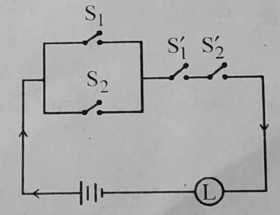Solution:

Let p,q denote the statements that the switches S1, S2 are closed respectively.

Let ~p, ~ q denote the statements that switch S1 and S2 are closed respectively. The symbolic form of the given switching circuit is (p v q) and (~p and ~q).

The switching table for the given switching circuit is as follows: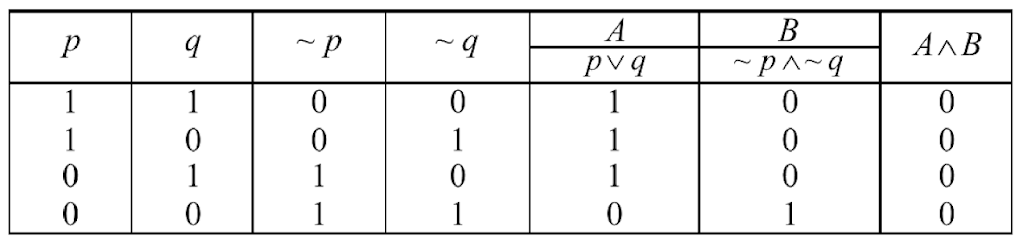In the last column of the switching table, each entry is zero, i.e. it is a contradiction. Hence, no current will flow in the circuit irrespective of the positions of the switches.

Question 22: If three numbers are added, their sum is 2. If two times the second number is subtracted from the sum of first and third numbers we get 8 and if three times the first number is added to the sum of second and third numbers we get 4. Find the numbers using matrices.

Solution:

Let the three numbers be x, y and z.

x + y + z = 2 —- (1)

(x + z) – 2y = 8

x – 2y + z = 8 —- (2)

3x + y + z = 4 —- (3)

Therefore,

x + y + z = 2 —- (1)

x – 2y + z = 8 —- (2)

3x + y + z = 4 —- (3)

The matrix form of these equations are

$$\begin{array}{l}\begin{pmatrix} 1 &1 &1 \\ 1&-2 &1 \\ 3&1 &1 \end{pmatrix}* \begin{pmatrix} x\\ y\\ z \end{pmatrix}=\begin{bmatrix} 2\\ 8\\ 4 \end{bmatrix}\end{array}$$

On applying the row operations,

x + y + z = 2 —- (1)

-3y = 6 —- (2)

– 2y – 2z = -2 —- (3)

From (2), y = -2

From (3),

– 2 * -2 – 2z = -2

4 – 2z = -2

6 = 2z

z = 3

x + y + z = 2

x – 2 + 3 = 2

x + 1 = 2

x = 1

The required numbers are 1, -2, 3.

Question 23: [a] In triangle ABC, with usual notations prove that b2 = c2 + a2 – 2a cos B.

OR

[b] In triangle ABC, with usual notations prove that (a – b)2 cos2 (C / 2) + (a + b)2 sin2 (C / 2) = c2.

Solution:

[a] Consider that for triangle ABC, ∠B is in a standard position i.e. vertex B is at the origin and the side BC is along the positive x-axis. As ∠B is an angle of a triangle, ∠B can be acute or ∠B can be obtuse.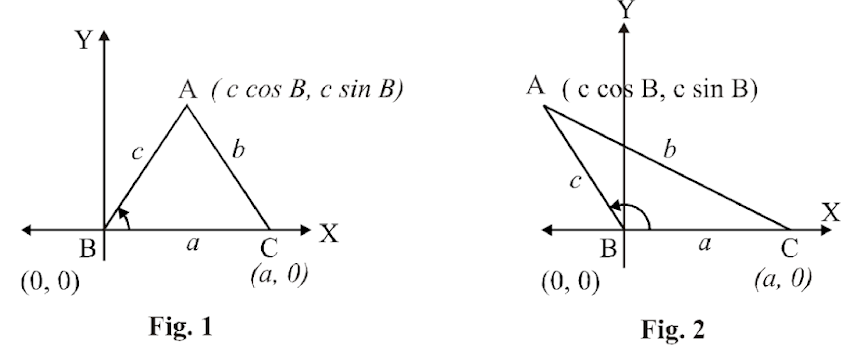Using the cartesian co-ordinate system in the above figure,

we get B ≣ (0,0) ; A ≣ (c cos B , c cos B) and C ≣ (a,0)

Now consider l (CA) = b

b2 = (a – c cos B)2 + (0 – c sin B)2

By distance formula,

b2 = a2 – 2 ac cos B + c2 cos2B + c2 sin2B

b2 = a2 – 2 ac cos B + c2 (sin2B + cos2B)

b2 = a2 + c2 – 2 ac cos B

[b] Taking LHS,

= (a – b)2 cos2 C / 2 + (a + b)2 sin2 C / 2

= (a2 + b2 – 2ab) cos2 C / 2 + (a2 + b2 + 2ab) . sin C / 2

= (a2 + b2) cos C / 2 – 2ab cos2 C / 2 + (a2 + b2) . sin2 C / 2 + 2ab sin2 C / 2

= (a2 + b2) (cos2 C / 2 + sin2 C / 2) – 2ab (cos2 C / 2 – sin2 C / 2)

= (a2 + b2) – 2ab cos C {By cosine Rule}

= c2

Question 24: Find ‘p’ and ‘q’ if the equation, px2 – 8xy + 3y2 + 14x + 2y + q = 0 represents a pair of perpendicular lines.

Solution:

Given general equation is px2 – 8xy + 3y2 + 14x + 2y + q = 0.

a = p , h = -4 , b = 3 , g = 7, f = 1 , c = q

Since the equation represents perpendicular lines;

a + b = 0

p + 3 = 0

p = -3;

a = p = -3

Since the equation represents a pair of lines.

-3 (3q – 1) + 4 (- 4q – 7) + 7 (- 4 – 21) = 0

-9q + 3 – 16q – 28 – 175 = 0

-25q – 200 = 0

-25q = 200

q = -8

p = -3 , q = -8

Question 25: Maximise: z = 3x + 5y subject to

x + 4y ≤ 24, 3x + y ≤ 21, x + y ≤ 9, x ≥ 0, y ≥ 0.

Solution: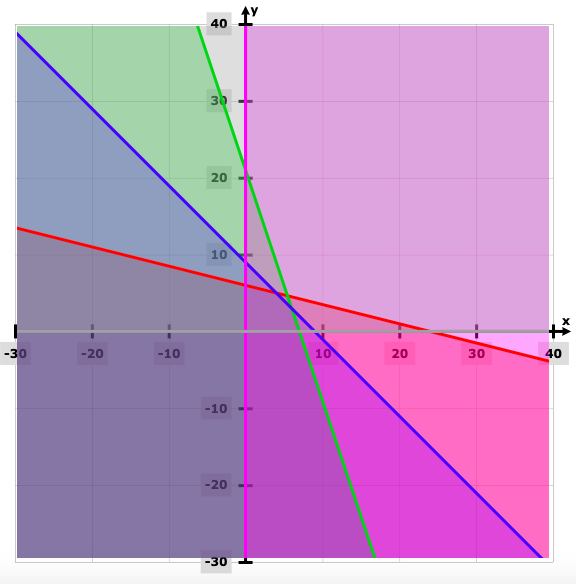Endpoints Value of z = 3x + 5y (0, 6) 30 (0,0) 0 (7, 0) 21 (6, 3) 33 (4, 5) 37

Z is maximum at the point (4, 5).

Question 26: If x = f(t) and y = g(t) are differentiable functions of t, then prove that y is a differentiable function of x and

dy / dx = (dy / dt) / (dx / dt), where dx / dt ≠ 0.

Hence, find dy / dt if x = acos2 t and y = asin2 t.

Solution:

Let δx and δy be the increments in x andy y, respectively, corresponding to the increment δt in t.

Since x and y are differentiable functions of t,

dx / dt = lim(δt→0) (δx) / (δx) and dy / dt = lim (δt→0) (δy) / (δt)…(1)

Also, as (δt→0), (δx→0) … (2)

Now , (δy) / (δx) = (δy) / (δt) / (δx) / (δt) …. (δt ≠ 0)

Taking limits as (δt→0),

lim(δt→0) (δy) / (δx) = lim(δt→0) (δy) / (δt) / (δx) / (δt) as (δt ≠ 0)

Taking limits as (δt→0),

lim(δt→0) (δy) / (δx) = lim(δt→0) (δy) / (δt) / (δx) / (δt)

lim(δx→0) (δy) / (δx) = (lim(δt→0) (δy) / (δt) / (lim(δx→0) (δx) / (δt)

= (dy / dt) / (dx / dt) ….[ By (1) and (2)]

∵ The limits in RHS exist.

lim(δx→0) (δy) / (δx) exists and is equal to dy / dx.

dy / dx = (dy / dt) / (dx / dt) if dx / dt ≠ 0

Hence

x = a cos2t

dx / dt = 2acost (-sint)

y = a sin2t

dy / dt = 2 a sint cos t

dy / dx = (dy / dt) / (dx / dt)

= 2a sint cost / -2a cost sint

= -1

Question 27: [a] f (x) = (x – 1) (x – 2) (x – 3), x ⥺ [0, 4], find ‘c’ if LMVT can be applied.

OR

[b] A rod of 108 meters long is bent to form a rectangle. Find its dimensions if the area is maximum.

Solution:

[a] f (x) = (x – 1) (x – 2) (x – 3) , x ⥺ [0, 4],

f (x) = x3 – 6x2 + 11x – 6

As f (x) is a polynomial in x,

(i) f (x) is continuous on [0, 4].

(ii) f (x) is differentiable on (0, 4).

Thus, all the conditions of LMVT are satisfied.

To verify LMVT we have to find c & c ⥺ (0, 4), such that

f’ (c) = f (4) – f (0) / 4 – 0 ……..(1)

f (4) = (4 – 1) (4 – 2) (4 – 3) = 6

f (0) = (0 – 1) (0 – 2) (0 – 3) = -6 and

f ‘ (x) = 3x2 – 12x + 11

f ‘ (c) = 3x2 – 12x + 11

From (1),

f (4) – f (0) / 4 – 0 = 3c2 – 12c + 11

(6 – (-6)) / 4 = 3c2 – 12c + 11

3 = 3c2 – 12c + 11

3c2 – 12c + 8 =0

c = 12 ± √(144 – 96)) / 6

c = 12 ± √48) / 6

c = 2 ± [2 / 3] √3

c = 2 ± 2 / √3

Both the value of c lie between 0 and 4.

OR

[b] 2x + 2y = 108

y = 54 – x

Now, area of rectangle = xy = x (54 – x)

f (x) = 54x – x2

f ‘ (x) = 54 – 2x

f ” (x) = -2

For extreme values f ‘( x) = 0

54 – 2x = 0

x = 27

f ” (27) = -2 < 0

Area is maximum when x =27 , y = 27

The dimensions of rectangle are 27m * 27m.

It is a square.

Question 28: Prove that: ∫dx / √x2 + a2 = log |x + √x2 + a2 | + c.

Solution:

Let I = ∫ 1 / √x2 + a2 dx

Put x = a tan 𝛉

tan 𝛉 = x / a

dx = a sec2 𝛉 d𝛉

I = ∫ 1/ √(a2 tan2 𝛉 + a2) asec2 𝛉 d 𝛉

= ∫ (a . sec2 𝛉) / (a √(1 + tan2 𝛉)) d𝛉

= ∫ (sec2 𝛉) / (sec 𝛉) d𝛉

= ∫ sec 𝛉 . d 𝛉

= log |sec 𝛉 + tan 𝛉| + c1

= log |x / a + √(sec2 𝛉)| + c1

= log |x / a + √ 1 + tan2 𝛉 | + c1

= log |x / a + √(1 + x2 / a2)| + c1

= log |x / a + √(a2 + x2) / a| + c1

= log |x + √(x2 + a2)| – log a + c1

∫dx / √x2 + a2 = log |x + √x2 + a2 | + c, where c = – log a +c

Question 29: Show that: ∫0𝛑/4 log (1 + tan x) dx = 𝛑 / 8 log 2.

Solution: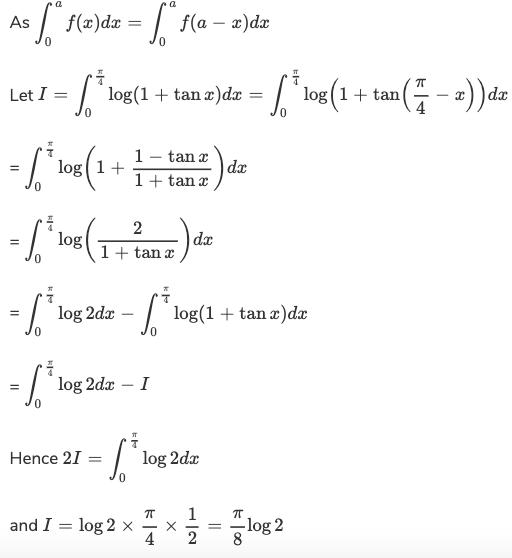Question 30: [a] Solve the differential equation (dy / dx) + y sec x = tan x.

OR

[b] Solve the differential equation (x + y) dy / dx = 1.

Solution:

[a] P = secx Q = tan x

IF = e∫sec xdx = e log(sec x + tan x) = secx + tanx

y * IF = ∫Q * IF

y (sec + tan x) = ∫(sec x + tan x) tan x.dx

= ∫sec x tan x + ∫ tan2 x dx

= secx + ∫(sec2 x – 1) dx

y(sec x + tan x) = sec x + tan x – x + c

[b] (x + y) dy / dx = 1

dy / dx = 1 / x + y

dx / dy = x + y

dx / dy + (-1) x = y

Comparing with dx / dy + P * x = Q,

P = -1, Q = y

= e∫-1ydy = e-y

The general solution is

x (IF) = ∫Q * IF dy + c

xe-y = y ∫e-y dy + c

xe-y = -ye-y – e-y + C

(x + y + 1) = cey